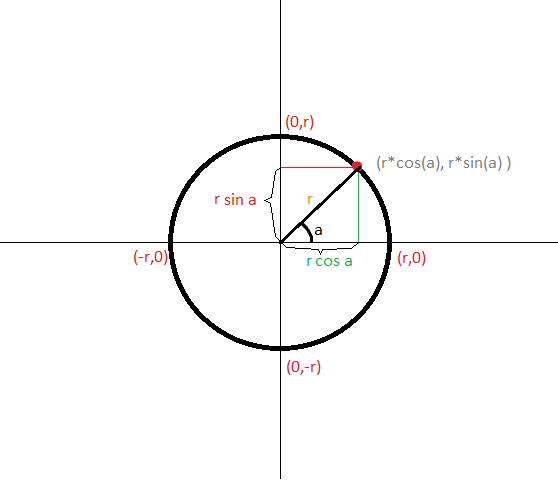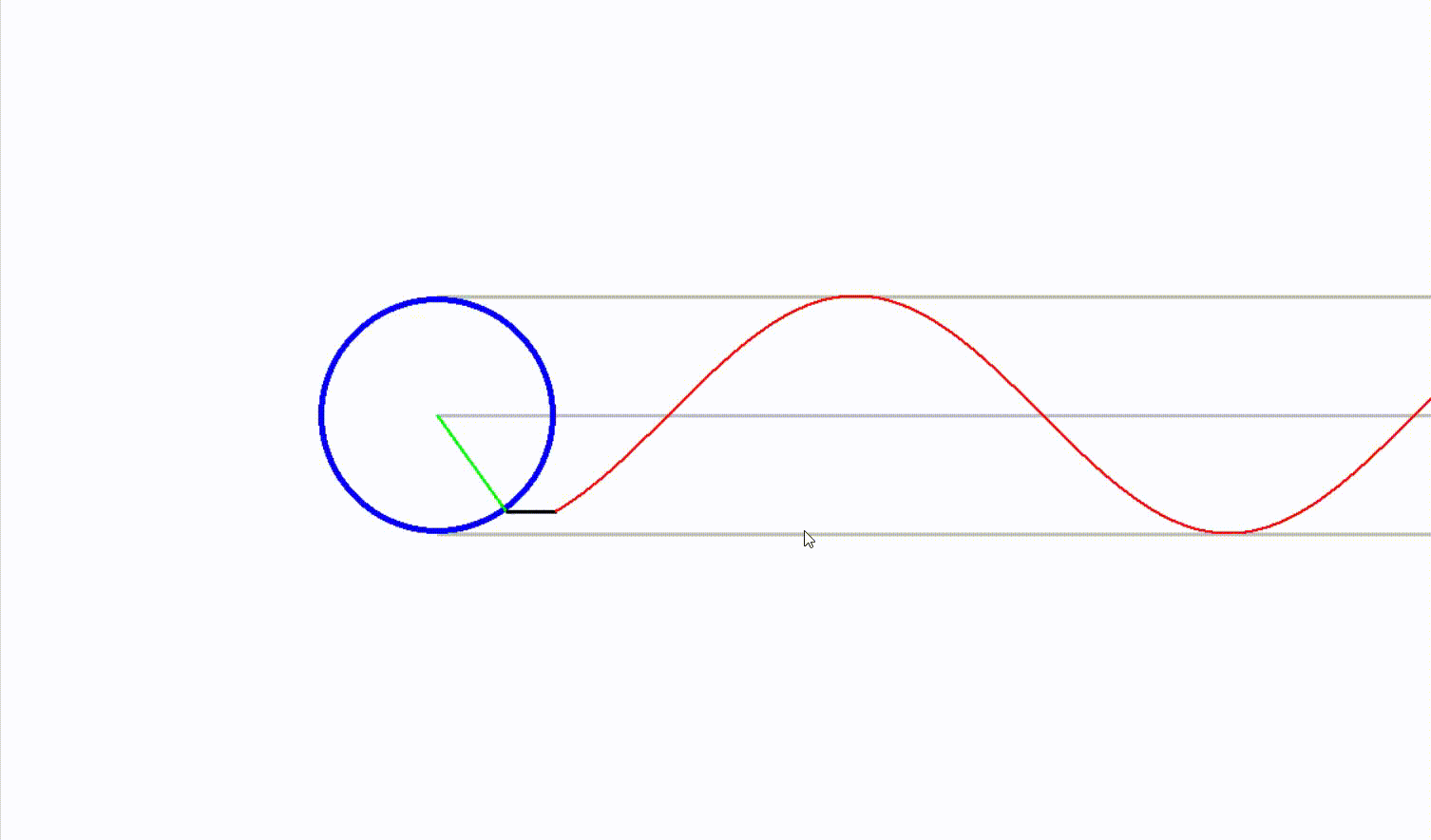Open in App
Not now

# Visualize sinusoidal waves using Python

• Last Updated : 16 Sep, 2021

Sinusoidal waves are the most basic trigonometric periodic curves, which is also known as the sine curve. Here we will see how sine function is related to a circle. Even though the sine function is a trigonometric function, it has more to do with a circle rather than a triangle.

Consider a simple equation of a circle:where r is the radius of the circle with center at origin (0,0) as shown in the figure below.

Sine is nothing but the measurement of the y-displacement from the origin as the angle increases as shown in the figure below:We can visualize this definition of sine using python and the pygame module. Pygame is an open-source python package that is mainly used for creating video games.

### Approach:

• Create a circle with radius r.
• Draw the radius of the circle. The endpoints of the radius will be (0,0) and (r*cos a, r*sin a), where the point (r*cos a, r*sin a) will always be of the circle.
• Draw the sine curve
• Then draw a line that will join the starting point of the sine wave and the endpoint of the radius of the circle. The length is called the gap for sake of simplicity.

Note: Here, the position of radius means the position of the head of the radius of the circle. Abs

## Drawing the sine curve

Draw a circle and animate its radius such that the endpoint of the radius will cover all the points on the circumference of the circle. This can be done using an infinite while loop and drawing a line from the center of the circle to (r* cos t,r*sin t). Then declare a list Ys to store all the r*sin t values at the beginning of the list.

This will basically keep a track of all the r*sin t values from the initial position of the radius till its current new position. These values will be used later to show the sine curve. Create a for loop to loop through the elements of Ys and then draw a circle of radius 1 and width 1, with abscissa starting from 0 to len(Ys) and ordinate will be the corresponding Ys values i.e, Ys[i], where index i[0, len(Ys)-1].  The abscissa needs to be shifted by a certain amount enough to make the animation tidy.

In the animation, the gap is shown by a black line that can be increased or decreased by the user.

## Python3

 `import` `numpy as np``import` `pygame``from` `pygame.``locals` `import` `*`  `class` `trig:` `    ``# to collect all the ordinates``    ``Ys ``=` `[]` `    ``def` `__init__(``self``, width``=``1600``, height``=``900``,``                 ``gap``=``100``, fps``=``60``, radius``=``100``):` `        ``# width of the window``        ``self``.width ``=` `width` `        ``# height of the window``        ``self``.height ``=` `height` `        ``# frame rate per second``        ``self``.fps ``=` `fps``        ``self``.screen ``=` `pygame.display.set_mode((``self``.width,``                                               ``self``.height))` `        ``# setting the screen dimensions``        ``self``.clock ``=` `pygame.time.Clock()` `        ``# the distance between the radius``        ``self``.gap ``=` `gap` `        ``#  pointer and the starting point of the curve` `        ``# the will be the x axis``        ``self``.t ``=` `0` `        ``# length of the radius of the circle``        ``self``.r ``=` `radius` `        ``self``.run ``=` `True``        ``while` `self``.run:``            ``self``.clock.tick(``self``.fps)` `            ``# filling the whole canvas with white background``            ``self``.screen.fill(``'white'``)``            ``for` `event ``in` `pygame.event.get():``                ``if` `event.``type` `=``=` `pygame.QUIT:``                    ``self``.run ``=` `False``            ``if` `event.``type` `=``=` `KEYDOWN:``                ``if` `event.key ``=``=` `K_ESCAPE:``                    ``pygame.quit()` `            ``# center of the circle``            ``x, y ``=` `400``, ``400` `            ``x ``+``=` `(``self``.r ``*` `np.cos(``self``.t))``            ``y ``+``=` `(``self``.r ``*` `np.sin(``self``.t))``            ``pygame.draw.line(``self``.screen, ``'grey'``,``                             ``(``400``, ``400``),``                             ``(``400``+``1000``, ``400``), ``3``)` `            ``# this will create a horizontal line` `            ``pygame.draw.line(``self``.screen,``                             ``'grey'``,``                             ``(``400``, ``400` `+` `self``.r),``                             ``(``400``+``1000``, ``400``+``self``.r), ``3``)` `            ``# this will create a horizontal line above the circle``            ``pygame.draw.line(``self``.screen,``                             ``'grey'``,``                             ``(``400``, ``400` `-` `self``.r),``                             ``(``400``+``1000``, ``400``-``self``.r),``                             ``3``)` `            ``# this will create a horizontal``            ``# line below the circle``            ``pygame.draw.circle(``self``.screen,``                               ``'blue'``,``                               ``(``400``, ``400``),``                               ``self``.r, ``5``)` `            ``# this will create a circle``            ``# with center (400,400)``            ``pygame.draw.line(``self``.screen,``                             ``'green'``,``                             ``(``400``, ``400``),``                             ``(x, y), ``3``)` `            ``# this will draw the radius of the circle` `            ``# inserting the y values``            ``# at the beginning of the Ys list``            ``self``.Ys.insert(``0``, y)` `            ``if` `len``(``self``.Ys) > ``1100` `-` `self``.gap:``                ``self``.Ys.pop()` `            ``# this will restrict the length``            ``# of the Ys to a certain limit``            ``# so that the animation``            ``# doesn't get out of the screen` `            ``pygame.draw.line(``self``.screen, ``'black'``, (x, y),``                             ``(``400``+``self``.gap, ``self``.Ys[``0``]), ``3``)` `            ``# this will create the joining line``            ``# between the curve and the circle's radius` `            ``for` `i ``in` `range``(``len``(``self``.Ys)):``                ``pygame.draw.circle(``self``.screen, ``'red'``,``                                   ``(i``+``400``+``self``.gap, ``self``.Ys[i]), ``1``, ``1``)` `                ``# this will create the sin curve``                ``# it will create bunch of small circles``                ``# with varying centers in such a``                ``# way that it will trajectory of``                ``# the centers of all those small circles``                ``# will give rise to a sine curve` `            ``if` `event.``type` `=``=` `KEYDOWN:``                ``if` `event.key ``=``=` `K_RIGHT:``                    ``self``.gap ``+``=` `1``                ``if` `event.key ``=``=` `K_LEFT:``                    ``self``.gap ``-``=` `1` `             ``# this part of code gives the user``             ``# the freedom to set the speed of the``             ``# animation and also set the gap``             ``# between the circle and the sine curve` `            ``self``.t ``+``=` `0.01``            ``pygame.display.update()`  `if` `__name__ ``=``=` `'__main__'``:``    ``sin ``=` `trig()``    ``pygame.quit()`

Output:Note: Use left and right arrows to decrease or increase the gap between the circle and the sine curve.  Use ECS  to exit the window or QUIT.

My Personal Notes arrow_drop_up